Fascinating Triangular Numbers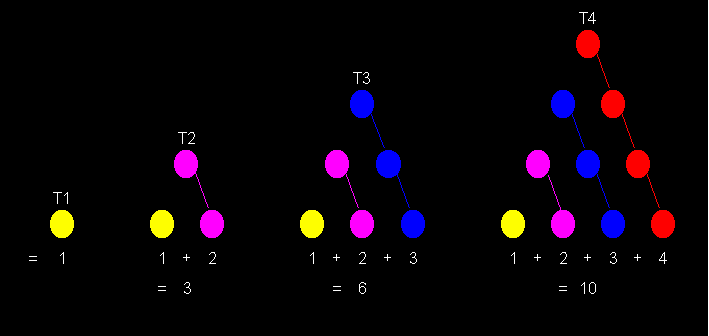The numbers which can be arranged in a compact triangular pattern are termed as triangular numbers. The triangular numbers are formed by partial sum of the series 1+2+3+4+5+6+7......+n. So

T1 = 1
T2 = 1 + 2 = 3
T3 = 1 + 2 + 3 = 6
T4 = 1 + 2 + 3 + 4 = 10

So the nth triangular number can be obtained as Tn = n*(n+1)/2, where n is any natural number.In other words triangular numbers form the series 1,3,6,10,15,21,28.....

Flocks of birds often fly in this triangular formation. Even several airplanes when flying together constitute this formation. The properties of such numbers were first studied by ancient Greek mathematicians, particularly the Pythagoreans.

Have you heard of the following famous story about the famous mathematician Carl F. Gauss.

" The teacher asked everyone in the class to find the sum of all the numbers from 1 to 100. To everybody's surprise, Gauss stood up with the answer 5050 immediately. The teacher asked him as to how it was done. Gauss explained that instead of adding all the numbers from 1 to 100, add first and last term i.e. 1 + 100 =101, then add second and second last term i.e. 2 + 99 =101 and so on. Every pair sum is 101 and their will be 50 such pairs ( total 100 numbers in all to be added), so 101 * 50 = 5050 is the answer. So the sum of numbers from 1 to N is (N/2)*(N+1), where N/2 are the number of pairs and N+1 is sum of each pair. This the famous formula for nth triangular number."

Some of the interesting properties of triangular numbers published in  are:

Curious properties of Triangular Numbers:

• The sum of two consecutive triangular numbers is always a square:

T1 + T2 = 1 + 3 = 4 = 22
T2 + T3 = 3 + 6 = 9 = 32

• If T is a Triangular number than 9*T + 1 is also a Triangular number:

9*T1 + 1 = 9 * 1 + 1 = 10 = T4
9*T2 + 1 = 9 * 3 + 1 = 28 = T7

• A Triangular number can never end in 2, 4, 7 or 9:
• If T is a Triangular number than 8*T + 1 is always a perfect square:

8*T1 + 1 = 8 * 1 + 1 = 9 = 32
8*T2 + 1 = 8 * 3 + 1 = 25 = 52

• The digital root (i.e. ultimate sum of digits until a single digit is obtained) of triangular numbers is always 1,3,6 or 9.
• The sum of n consecutive cubes starting from 1 is equal to the square of nth triangular number i.e. Tn2 = 13 + 23 + 33 + ... + n3

T42 = 102 = 100 = 13 + 23 + 33 + 43
T52 = 152 = 225 = 13 + 23 + 33 + 43 + 53

• A triangular number greater than 1, can never be a Cube, a Fourth Power or a Fifth power.
• There do not exist four distinct triangular numbers in arithmetic progression.
• There do not exist four distinct triangular numbers in geometric progression.
• The sum of the squares of two consecutive triangular numbers is also a triangular number.

T12 + T22 = 1 2 + 3 2= 10 = T4
T22 + T32 = 3 2 + 6 2= 45 = T9
T32 + T42 = 6 2 + 10 2= 136 = T16

Tn-12 + Tn2 = Tn2

(Dr. Diego Marques, University of Brasilia, Brazil submitted vide his email dated 20 Apr 2011 that "this is also valid for the amazing Fibonacci sequence i.e. The sum of the squares
of two consecutive fibonacci numbers is also a fibonacci number).

• All perfect numbers are triangular numbers.
• The square of triangular numbers 1 and 6 produce triangular numbers 1 and 36.

T12 = 1 * 1 = 1 = T1
T32 = 6 * 6 = 36 = T8

Can anybody find the third triangular number whose square is also a triangular number ?.

(AMRIK S NIMBRAN from Patna, Bihar submitted vide his email dated 21 Dec 2011 that "It took me some time to locate the proof of the impossibility of any other square triangular number
beside 1 and 36 which is square of a triangular number, The source of proof is: L. J. Mordel, Diophantine Equations, 1969, Academic Press, London, Theorem 7, pp. 268-269).

• The numbers in the sequence 1, 11, 111, 1111, 11111,...etc. are all triangular numbers in base 9.
• The only triangular number which is also a prime is 3.
• Palindromic Triangular Numbers:Some of the many triangular numbers, which are also palindromic ( i.e. reading the same forward as well as backward) are 1, 3, 6, 55, 66, 171, 595, 666, 3003, 5995, 8778, 15051, 66066, 617716, 828828, 1269621, 1680861, 3544453, 5073705, 5676765, 6295926, 351335153, 61477416, 178727871, 1264114621, 1634004361 etc. These can be termed as palindromic triangular numbers. There are 28 palindromic Triangular numbers below 1010.

The largest known palindromic triangular number containing only odd digits:
T32850970 = 539593131395935

The largest known palindromic triangular number containing only even digits:
T128127032 = 8208268228628028

For more on these numbers visit Patrick De Geest
• Reversible Triangular Numbers: Some of the many triangular numbers, whose reversals are also triangular numbers are:

1,3,6,10,55,66,120,153,171,190,300,351,595,630,666,820,3003,5995,8778,15051,
17578,66066,87571,156520,180300,185745,547581,557040,617716,678030,828828,
1269621,1461195,1680861,1851850,3544453,5073705,5676765,5911641,6056940,
6295926,12145056,12517506,16678200,35133153,56440000,60571521,61477416,
65054121,157433640,178727871,188267310,304119453,354911403,1261250200,
1264114621,1382301910,1634004361,1775275491,1945725771 etc.

These can be termed as Reversible triangular numbers.
Note that all palindromic triangular numbers mentioned above are special case of reversible triangular numbers.
• Square Triangular Numbers: There are infinitely many triangular numbers, which are also squares as given by the series 1, 36, 1225, 41616, 1413721, 48024900, 1631432881, 55420693056 etc. These can be termed as Square triangular(ST) numbers. The nth Square Triangular number Kn can easily be obtained from the recursive formula

Kn = 34 * Kn-1 - Kn-2 + 2.

So knowing the first two ST numbers i.e. K1 = 1 and K2 = 36 , all other successive Square Triangular numbers can be obtained , e.g.

K3 = 34 * K2 - K1 + 2 = 34 * 36 -1 + 2 = 1225

K4 = 34 * K3 - K2 + 2 = 34 * 1225 - 36 + 2 = 41616

The following non- recursive formula also gives nth Square Triangular number in terms of variable n.

Kn = [{(1 + 2½)2n - (1 - 2½)2n}/(4*2½)]2

It is interesting to note that digital root of all EVEN Square Triangular Numbers i.e. 36, 41616, 48024900, 55420693056 .. etc is always 9 and digital root of all ODD Square Triangular Numbers i.e. 1, 1225, 1413721, 1631432881, ... etc is always 1. Also Square Triangular Numbers can never end in 2, 3, 4, 7, 8 or 9.

• There are pairs of triangular numbers such that the sum and difference of numbers in each pair are also triangular numbers e.g. (15, 21), (105, 171), (378, 703), (780, 990), (1485, 4186), (2145, 3741), (5460, 6786), (7875, 8778)... etc.:

21 + 15 = 36 = T8 : 21 - 15 = 6 = T3

171 + 105 = 276 = T23 : 171 - 105 = 66 = T11

703 + 378 = 1081 = T46 : 703 - 378 = 325 = T25

and so on.

• There exist infinite triangular numbers that are simultaneously the sum, the difference and the product of two triangular numbers, for example:

T55 = T10 + T54 = T1540 - T1539 = T7 * T10

T75 = T29 + T69 = T77 - T17 = T5 * T19

and so on.

Some New Observations on Triangular Numbers :

• There are some triangular numbers which are product of three consecutive numbers. For example 120 is a triangular number which is product of three consecutive numbers 5, 6 and 7.There are only 6 such triangular numbers, largest of which is 258474216, as shown below:

1 * 2 * 3 = 6 = T3

4 * 5 * 6 = 120 = T15

5 * 6 * 7 = 210 = T20

9 * 10 * 11 = 990 = T44

56 * 57 * 58 = 185136 = T608

636 * 637 * 638 = 258474216 = T22736

• The triangular number 120 is the product of three, four and five consecutive numbers.

4 * 5 * 6 = 2 * 3 * 4 * 5 = 1 * 2 * 3 * 4 * 5 = 120

No other triangular number is known to be the product of four or more consecutive numbers.

• There are infinite triangular numbers which are product of two consecutive numbers. For example 6 is a triangular number which is product of two consecutive numbers 2 and 3. Some others are as shown below:

2 * 3 = 6 = T3

14 * 15 = 210 = T20

84 * 85 = 7140 = T119

492 * 493 = 242556 = T696

2870 * 2871 = 8239770 = T4059

16730 * 16731 = 279909630 = T23660

97512 * 97513 = 9508687656 = T137903

568344 * 568345 = 323015470680 = T803760

3312554 * 3312555 = 10973017315470 = T4684659

19306982 * 19306983 = 372759573255306 = T27304196  etc.

• The triangular numbers which are product of two prime numbers can be termed as Triangular Semiprimes .
For example 6 is a Triangular Semiprimes . Some other examples of Triangular Semiprimes are:

10,15,21,55,91,253,703,1081,1711,1891,2701,3403,5671,12403,13861,15931,
18721,25651,34453,38503,49141,60031,64261,73153,79003,88831,104653,108811,
114481,126253,146611,158203,171991,188191,218791,226801,258121,269011,286903,
351541,371953,385003,392941,482653,497503 etc as shown below:

2 * 3 = 6 = T3

3 * 5 = 15 = T5

3 * 7 = 21 = T6

5 * 11 = 55 = T10

7 * 13 = 91 = T13

11 * 23 = 253 = T22

19 * 37 = 703 = T37

Harshad (or Niven ) numbers are those numbers which are divisible by their sum of the digits. For example 1729 ( 19*91) is divisible by 1+7+2+9 =19, so 1729 is a Harshad number.

Harshad Triangular Number can be defined as the Triangular numbers which are divisible by the sum of their digits. For example, Triangular number 1128 is divisible by 1+1+2+8 = 12 (i.e. 1128/12 = 94). So 1128 is a Harshad Triangular Number. Other examples are:

1, 3, 6, 10, 21, 36, 45, 120, 153, 171, 190, 210, 300, 351, 378, 465, 630, 666, 780, 820, 990, 1035, 1128, 1275, 1431, 1540, 1596, 1770, 2016, 2080, 2556, 2628, 2850, 2926, 3160, 3240, 3321, 3486, 3570, 4005, 4465, 4560, 4950, 5050, 5460, 5565, 5778, 5886, 7140, 7260, 8001, 8911, 9180, 10011, 10296, 10440, 11175, 11476, 11628, 12720, 13041, 13203, 14196, 14706, 15225, 15400, 15576, 16110, 16290, 16653, 17020, 17205, 17766, 17955, 18145, 18528, 20100, 21321, 21528, 21736, 21945, 22155, 23220, 23436, 24090, 24310, 24976, 25200, 28680, 29646, 30628, 31626, 32640, 33930, 35245, 36585, 37128, 39060, 40470, 41328, 41616, 43365, 43956, 45150, 46360, 51040, 51360, 51681, 52326, 52650, 53956, 56280, 56616, 61776, 63903, 64620, 65341, 67896, 69006, 70125, 70500, 72010, 73536, 73920, 76636, 78210, 79401, 79800, 80200,81810, 88410, 89676, 90100, 93096, 93528, 97020, 100128, 101025, 103740, 105111, 105570 etc.

• Happy Triangular Numbers:

If you iterate the process of summing the squares of the decimal digits of a number and if the process terminates in 1, then the original number is called a Happy number. For example 7 -> 49 -> 97 -> 130 -> 10 -> 1.

A Happy Triangular Number is defined as a Triangular number which is also a Happy number. For example, consider a triangular number 946, where 946 -> 133 -> 19 -> 82 -> 68 -> 100 -> 1. So 946 is a Happy triangular Number. Other examples of Happy Triangular Numbers are :

1, 10, 28, 91, 190, 496, 820, 946, 1128, 1275, 2080, 2211, 2485, 3321, 4278, 8128, 8256, 8778, 9591, 9730, 11476, 12090, 12880, 13203, 13366, 13530, 15753, 16471, 17205, 17578, 20910, 21115, 21321, 22791, 24753, 25651, 27261, 29890, 30135, 31626, 33670, 35245, 36046, 41328, 43660, 43956, 44253, 46360, 47586, 48205, 50721, 53301, 53956, 54615, 55278, 56280, 56953, 58311, 61425, 62128, 66430, 69378, 69751, 70125, 75855, 76245, 77815, 79003, 80200, 81810, 82621, 84666, 87571, 90100, 90951, 93961, 99681, 100128, 101025, 102831, 103285, 105570, 107416, 110215, 117370, 119316, 122760, 123256, 123753, 126253, 127260, 129286, 130305 etc.

• The only Fibonacci Numbers that are also triangular are 1, 3, 21 and 55.
• The only triangular Numbers that are also repdigit are 55, 66 and 666.
• 3 is the only triangular Number that is also a Fermat Number.
• Every Hexagonal number is a triangular number.
• Every pentagonal number is one-third of a triangular number.
• If M is a Mersenne prime then Mth triangular number is a perfect number.
• The sum of reciprocals of triangular numbers converges to 2:

1 + 1/3 + 1/6 + 1/10 + 1/15 + 1/21 + 1/28 + 1/36 + 1/45 + ....= 2
• There are many pairs of triangular numbers Ta and Tb (where Ta > 1 and Tb > 1 and Ta is not equal to Tb) such that their product Ta*Tb is a Perfect Square. For example:

T2 * T24 = 3 * 300 = 900 = 302

T2 * T242 = 3 * 29403 = 88209 = 2972

T3 * T48 = 6 * 1176 = 7056 = 842

T6 * T168 = 21 * 14196 = 298116 = 5462

T11 * T528 = 66 * 139656 = 9217296 = 30362

T12 * T624 = 78 * 195000 = 15210000 = 39002

Note: The product of Ta*Tb is a perfect square for the formula (2a + 1)2 - 1 = b. This produces a perfect square for EVERY triangular index and therefore every triangular number.

(Note submitted by Steve Homewood vide his email dated 27 June 2018).

• Every positive integer can be written as a sum of atmost three triangular numbers.
• Every 4th power greater than 1, is the sum of two triangular numbers.

24 = 16 = T3 + T4 = T1 + T5

34 = 81 = T8 + T9 = T5 + T11

44 = 256 = T15 + T16 = T11 + T19

54 = 625 = T24 + T25 = T19 + T29

64 = 1296 = T35 + T36 = T29 + T41

74 = 2401 = T48 + T49 = T41 + T55

Observe the patterns formed above.

Observation: If n4 = Ta + Tb = Tc + Td then a = n2 - 1, b = n2, c = a - n or n2 - n - 1 and d = a + b - c - 1 or n2 + n - 1.

(Observation submitted by Steve Homewood vide his email dated 1st July 2018).

• For any natural number n, the number 1 + 9 + 92 + 93 + ... + 9n is a triangular number.

1 = T1
1 + 9 = T4
1 + 9 + 92 = T13
1 + 9 + 92 + 93 = T40
1 + 9 + 92 + 93 + 94 = T121
• A curious pattern :

T1 + T2 + T3= T4
T5 + T6 + T7 + T8 = T9 + T10
T11 + T12 + T13 + T14 + T15= T16 + T17 + T18
T19 + T20 + T21 + T22 + T23 + T24 = T25 + T26 + T27 + T28

• Some identities :
Tn2 = Tn + Tn-1 * Tn+1
Tn2-1 = 2*Tn * Tn-1
(2*a + 1)2 * Tn + Ta = T(2*a + 1)* n + a

For more on such identities refer Terry Trotter .
• Triangular numbers appear in Pascal's Triangle. In fact 3rd diagonal of Pascal's Triangle, gives all triangular numbers as shown below:

1
1 1
1 2 1
1 3 3 1
1 4 6 4 1
1 5 10 10 5 1
1 6 15 20 15 6 1
1 7 21 35 35 21 7 1
1 8 28 56 70 56 28 8 1
1 9 36 84 126 126 84 36 9 1

• The only known example of a Pythagorean triangle (a,b,c) where a, b, c are triangular numbers is (8778, 10296, 13530):

87782 + 102962 = 135302

(T132)2 + (T143)2 = (T164)2

The only known examples of a Pythagorean triangle such that both Perimeter as well as Area are triangular numbers are:

(3312, 14091, 14475) with Perimeter = 31878 = T252 and Area = 23334696 = T6831

(3405996, 8013265, 8707079) with Perimeter = 20126340 = T6344 and Area = 13646574268470 = T5224284

Can you find other examples ? For more visit Carlos Rivera.

Observe the amazing curiosity in the following Pythagorean triplets which are all generated by the Pythagorean triplet (5, 12, 13) by prefixing triangular numbers:
15, 112, 113
25, 312, 313
35, 612, 613
45, 1012, 1013
55, 1512, 1513 and so on.
This can be generalised as follows (where 5 is concatenated with n and, 12, 13 are concatenated with nth triangular numbers):
[n5]2 + [Tn12]2 = [Tn13]2.

(submitted by Julian Beauchamp vide his email dated 24 November 2022).

• T8 + T35 + T23 = T9 + T21 + T36

8  +  35   +  23 =  9  +  21  +  36

• Sum of Consecutive Triangular Numbers:

T28 + T29 + T30 + ... + T34 = T83

T118 + T119 + T120 + ... + T124 = T321

T16 + T17 + T18 + T19 = 2*T25

T103 + T104 + T105 + T106 = 2*T148

• Observe this pattern of infinite triangular numbers.

T10 = T5+5 = 55

T100 = T50+50 = 5050

T1000 = T500+500 = 500500

T10000 = T5000+5000 = 50005000

T100000 = T50000+50000 = 5000050000

T1000000 = T500000+500000 = 500000500000     and so on.

• TT5 + TT6 = T26

TT12 + TT14 = T131

TT15 + TT42 = T911

TT26 + TT34 = T691

TT36 + TT55 = T1678

TT42 + TT120 = T7316     and so on.

• Concatenated Triangular numbers:

13 and 136101521 are primes formed by concatenation of first n triangular numbers. 136 is the only known triangular number formed from concatenation of first n triangular numbers. 31, 631, 10631, 55453628211510631, 786655453628211510631 and 10591786655453628211510631 are primes formed by reverse concatenation of first n triangular numbers .

• Highly Composite Triangular Numbers:

Numbers such that d(n), the number of divisors of n, is greater than for any smaller n are called highly composite numbers. If n is a triangular number then it can be termed as Highly Composite Triangular Number . For example 28 is a triangular number and d(28) = 6 . Number of divisors of all triangular numbers less than 28 is less than 6. So 28 is a Highly Composite Triangular number.
All Highly Composite Triangular numbers below 5*1013 are:

1, 3, 6, 28, 36, 120, 300, 528, 630, 2016, 3240, 5460, 25200, 73920, 157080, 437580, 749700, 1385280, 1493856, 2031120, 2162160, 17907120, 76576500, 103672800, 236215980, 842161320, 3090906000, 4819214400, 7589181600, 7966312200, 13674528000, 20366564400, 49172323200, 78091322400, 102774672000, 557736444720, 666365279580, 876785263200, 1787835551040, 2427046221600, 3798207594720, 24287658595200 and 26571463158240.

• Abundant and Deficient Triangular Numbers:

Numbers such that s(n), the sum of aliquot divisors of n, is greater than n are called Abundant numbers. If n is a triangular number then it can be termed as Abundant Triangular Number . For example 36 is a triangular number and s(36) = 1 + 2 + 3 + 4 + 6 + 9 + 12 + 18 = 55, which is greater than 36. So 36 is a Abundant Triangular number.
All Abundant Triangular numbers below 105 are:

36,66,78,120,210,276,300,378,528,630,666,780,820,990,1128,1176,1326,1540,1596, 1770,1830,2016,2080,2346,2556,2628,2850,3160,3240,3486,3570,3828,4095,4278, 4560,4656,4950,5460,5778,5886,6216,6328,6786,7140,7260,7626,7875,8256,8646, 8778,9180,9730,9870,10296,10440,10878,11628,12090,12246,12720,12880,13530, 14028,14196,14706,15400,15576,16110,16290,16836,17020,17766,17955,18336,18528, 19110,19900,20100,20706,20910,21528,21736,21945,22578,23220,23436,24090,24310, 24976,25200,25878,26106,26565,26796,27966,28680,28920,29646,29890,30628,30876, 31626,31878,32640,33670,33930,34716,34980,35778,37128,37950,38226,39060,39340, 40470,41328,41616,42486,43660,43956,44850,45150,46056,46360,47586,48516,48828, 49770,51040,51360,52326,52650,53628,53956,54285,55278,56280,56616,57630,57970, 58996,59340,60378,60726,61425,61776,62128,63546,64620,64980,66066,66430,67896, 69006,69378,70500,72390,73536,73920,75078,76636,77028,78210,78606,79800,80200, 81810,82215,83028,83436,84666,86320,86736,87990,88410,89676,90100,91806,93096, 93528,94830,96580,97020,98346 and 98790.

Numbers such that s(n), the sum of aliquot divisors of n, is less than n are called Deficient numbers. If n is a triangular number then it can be termed as Deficient Triangular Number . For example 21 is a triangular number and s(21) = 1 + 3 + 7 = 11, which is less than 21. So 21 is a Deficient Triangular number.
All Deficient Triangular numbers below 105 are:

1,3,10,15,21,45,55,91,105,136,153,171,190,231,253,325,351,406,435,465, 561,595,703,741,861,903,946,1035,1081,1225,1275,1378,1431,1485,1653,1711, 1891,1953,2145,2211,2278,2415,2485,2701,2775,2926,3003,3081,3321,3403,3655, 3741,3916,4005,4186,4371,4465,4753,4851,5050,5151,5253,5356,5565,5671,5995, 6105,6441,6555,6670,6903,7021,7381,7503,7750,8001,8385,8515,8911,9045, 9316,9453,9591,10011,10153,10585,10731,11026,11175,11325,11476,11781,11935, 12403,12561,13041,13203,13366,13695,13861,14365,14535,14878,15051,15225,15753, 15931,16471,16653,17205,17391,17578,18145,18721,18915,19306,19503,19701,20301, 20503,21115,21321,22155,22366,22791,23005,23653,23871,24531,24753,25425,25651, 26335,27028,27261,27495,27730,28203,28441,29161,29403,30135,30381,31125,31375, 32131,32385,32896,33153,33411,34191,34453,35245,35511,36046,36315,36585,36856, 37401,37675,38503,38781,39621,39903,40186,40755,41041,41905,42195,42778,43071, 43365,44253,44551,45451,45753,46665,46971,47278,47895,48205,49141,49455,50086, 50403,50721,51681,52003,52975,53301,54615,54946,55611,55945,56953,57291,58311, 58653,59685,60031,61075,62481,62835,63190,63903,64261,65341,65703,66795,67161, 67528,68265,68635,69751,70125,70876,71253,71631,72010,72771,73153,74305,74691, 75466,75855,76245,77421,77815,79003,79401,80601,81003,81406,82621,83845,84255, 85078,85491,85905,87153,87571,88831,89253,90525,90951,91378,92235,92665,93961, 94395,95266,95703,96141,97461,97903,99235 and 99681.

• Curious Sum of Two Triangular Numbers.

Take an example of a 10-digit Triangular number 1061444835. It can be seen that this triangular number is the sum of the 10614th and 44835th triangular numbers. So the sum of two triangular numbers is equal to the number formed from concatenation of index of these two triangular numbers.

T10614 + T44835 = 1061444835

Other examples are:

T90 + T415 = 90415

T585 + T910 = 585910

T120 + T1545 = 1201545

T150 + T1726 = 1501726

T244 + T2196 = 2442196

T700 + T3676 = 7003676

T769 + T3846 = 7693846

T1474 + T5226 = 14745226

T2829 + T6970 = 28296970

T3030 + T7171 = 30307171     and so on.

Can you observe beautiful pattern in last two examples.

• Observe more patterns of infinite triangular numbers.

T6 = 21

T66 = 2211

T666 = 222111

T6666 = 22221111      and so on.

(submitted by William Downie vide his email dated 9 Sept 2015).

• T3 = 6

T33 = 561

T333 = 55611

T3333 = 5556111      and so on.

• Sequence of sqrt(T) which are whole numbers is:

√ T1, √ T8, √ T49, √ T288, √ T1681, √ T9800, √ T57121,..

The product of any two consecutive numbers in above sequence is a triangular number, which is the product of two consecutive numbers:

√ T1 * √ T8 = 6 = T3 = 2 * 3

√ T8 * √ T49 = 210 = T20 = 14 * 15

√ T49 * √ T288 = 7140 = T119 = 84 * 85

√ T288 * √ T1681 = 242556 = T696 = 492 * 493

√ T1681 * √ T9800 = 8239770 = T4059 = 2870 * 2871

√ T9800 * √ T57121 = 279909630 = T23660 = 16730 * 16731

and so on.

(submitted by Dr. N Mander (Mr) from Denmark vide his email dated 22 Oct 2011).

• There are infinite number of pairs (a,b) of positive integers such that 2 * Ta=Tb where a and b are integers and Tn is the nth triangular number.

2 * T2 = T3 = 6

2 * T14 = T20 = 210

2 * T84 = T119 = 7140

2 * T492 = T696 = 242556

2 * T2870 = T4059 = 8239770

2 * T16730 = T23660 = 279909630

2 * T97512 = T137903 = 9508687656

2 * T568344 = T803760 = 323015470680

2 * T3312554 = T4684659 = 10973017315470

2 * T19306982 = T27304196 = 372759573255306

and so on.

With a0 = 0 and b0 = 0 , the following recursive equations can give further values of a and b:

an=3 * an-1 + 2 * bn-1 + 2

bn=4 * an-1 + 3 * bn-1 + 3

(submitted by Mr Roy Blatchford vide his email dated 17 Mar 2011).

• There are infinite number of pairs (a,b) of positive integers such that 3 * Ta=Tb where a and b are integers and Tn is the nth triangular number.

3 * T1 = T2 = 3

3 * T5 = T9 = 45

3 * T20 = T35 = 630

3 * T76 = T132 = 8778

3 * T285 = T494 = 122265

3 * T1065 = T1845 = 1702935

3 * T3976 = T6887 = 23718828

3 * T14840 = T25704 = 330360660

3 * T55385 = T95930 = 4601330415

3 * T206701 = T358017 = 64088265153

and so on.

With a0 = 0 and b0 = 0 , the following recursive equations can give further values of a and b:

an = 2 * an-1 + bn-1 + 1

bn = 3 * an-1 + 2 * bn-1 + 2

(submitted by Dr. Muniru A. Asiru from Nigeria vide his email dated 28 Dec 2014).

• Cubes from Triangular Numbers :

6 * ( T1 + T2 + T3) + 4 = 43

6 * ( T1 + T2 + T3 + T4) + 5 = 53

6 * ( T1 + T2 + T3 + T4 + T5) + 6 = 63

6 * ( T1 + T2 + T3 + T4 + T5 + T6) + 7 = 73

6 * ( T1 + T2 + T3 + T4 + T5 + ...... + Tn-1) + n = n3

(submitted by Don Davis vide his email dated 20 June 2013).

• The square of a triangular number subtracted from the square of the next triangular number is a cube.

T22 - T12 = 23

T32 - T22 = 33

T42 - T32 = 43

T52 - T42 = 53

Tn2 - Tn-12 = n3

(submitted by Berke Terzioglu vide his email dated 27 May 2015).

• Interesting cube pattern with triangular numbers.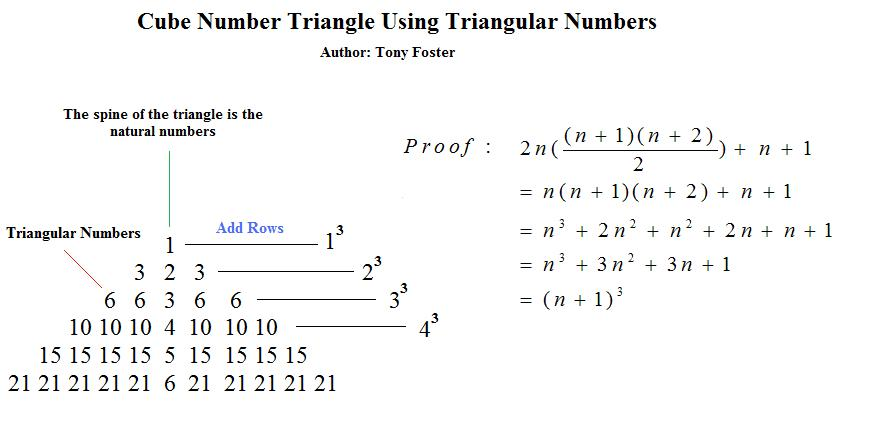(submitted by Tony Foster vide his email dated 24 July 2015).

• Connection Between Golden Ratio and Triangular Numbers.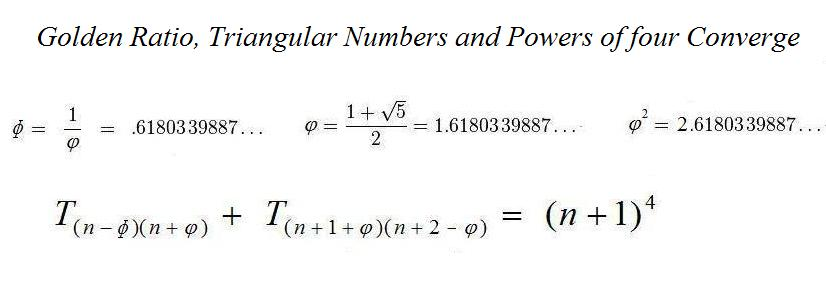(submitted by Tony Foster vide his email dated 29 July 2015).

• Sum of triangular numbers squared in pascals triangle.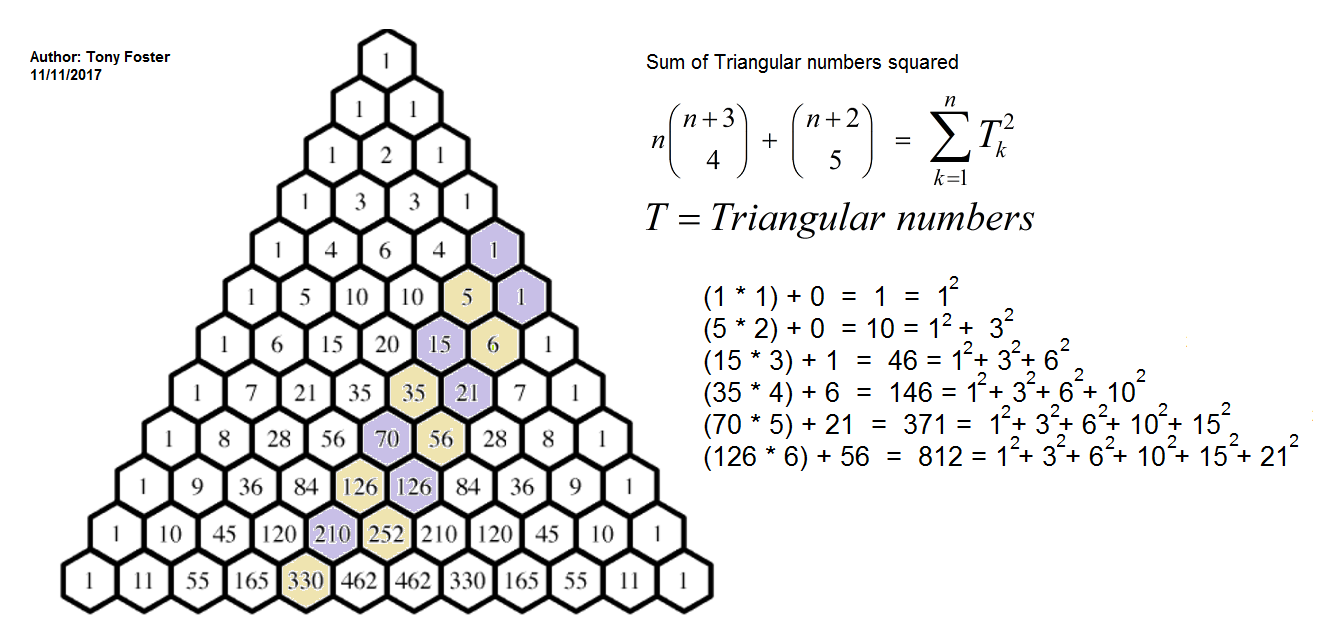(submitted by Tony Foster vide his email dated 20 November 2017).

• A neat triangular number pattern.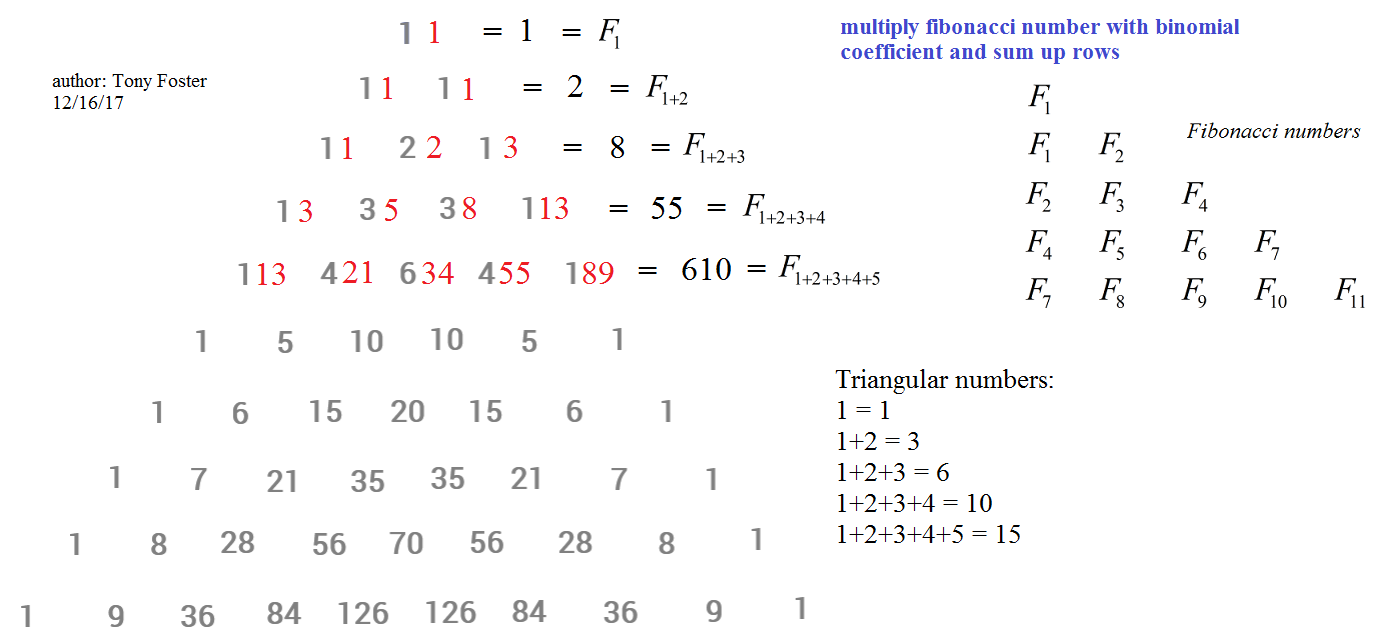(submitted by Tony Foster vide his email dated 17 December 2017).

• Relation Between π and Triangular Numbers.

The sum of reciprocals of squares of all the triangular numbers converges to 4*(π2 - 9)/3:

1 + 1/32 + 1/62 + 1/102 + 1/152 + 1/212 + 1/282 + 1/362 + 1/452 + ....= 4*(π2 - 9)/3

The sum of reciprocals of cubes of all the triangular numbers converges to 8*(10 - π2):

1 + 1/33 + 1/63 + 1/103 + 1/153 + 1/213 + 1/283 + 1/363 + 1/453 + ....= 8*(10 - π2)

(submitted by Okoh Ufuoma Cyrus from Nigeria vide his email dated 26 April 2013).

• The sum of reciprocals of triangular numbers with alternating signs is:

1 - 1/3 + 1/6 - 1/10 + 1/15 - 1/21 + 1/28 - 1/36 + 1/45 - ....= 4*log2 - 2 (about 0.7725887222)

The sum of reciprocals of squares of triangular numbers with alternating signs is:

1 - 1/32 + 1/62 - 1/102 + 1/152 - 1/212 + 1/282 - 1/362 + 1/452 - ....= 12 - 16*log2 (about 0.9096451110)

The sum of reciprocals of cubes of triangular numbers with alternating signs is:

1 - 1/33 + 1/63 - 1/103 + 1/153 - 1/213 + 1/283 - 1/363 + 1/453 - ....= 96*log2 + 12*zeta(3) - 80 (about 0.9668121717)

(submitted by Mauro Fiorentini vide his email dated 3 January 2015).

• The Sum of the Squares of consecutive Triangular Numbers:

T112 + T122 + T132 = T193

T12 + T22 + T32 + T42 + T52+ T62 + T72 = T56

T42 + T52 + T62 + T72 + T82+ T92 + T102 + T112 + T122 = T191

T12 + T22 + T32 + T42 +.......+ T232 + T242 + T252 + T262 = T1196

T12 + T22 + T32 + T42 +.......+ T862 + T872 + T882 + T892 = T24297

T742 + T752 + T762 + T772 +.......+ T1612 + T1622 + T1632 + T1642 = T109577

(submitted by John McMahon vide his email dated 3 May 2018 and 2 December 2018).

• The Sum of ten consecutive Triangular Numbers:

Starting with T0 = 0, in base 10,

The sum of the first 10 triangular numbers from T0 to T9 = 165
The sum of the next 10 triangular numbers from T10 to T19 = 1165
The sum of the next 10 triangular numbers from T20 to T29 = 3165
The sum of the next 10 triangular numbers from T30 to T39 = 6165
The sum of the next 10 triangular numbers from T40 to T49 = 10165
The sum of the next 10 triangular numbers from T50 to T59 = 15165
and so on.

The same pattern is evident in other bases. For example, in base 2

Starting with T0 = 0, in base 2,

The sum of the first 2 triangular numbers from T0 to T1 = 1
The sum of the next 2 triangular numbers = 1001
The sum of the next 2 triangular numbers = 11001
The sum of the next 2 triangular numbers = 110001
The sum of the next 2 triangular numbers = 1010001
The sum of the next 2 triangular numbers = 11111001
and so on.

Note that the number of triangular numbers to add must correspond to the base number itself. The last (three?) digits are always the same. The preceding digits are always the triangular numbers (in their respective bases).

(submitted by Julian Beauchamp vide his email dated 9 May 2019).

• Triangular Numbers and Sqrt 2:

There is a nice link between triangular numbers and √2 as given below:
as n -> infinity, √Tn+1 - √Tn = √2/2.

The proof is simple:
Using the general formula for triangular numbers, Tn = n(n+1), we get,
√ [(n+1)(n+2)/2] - √[n(n+1)/2] = √2/2.
=> √ [(n+1)(n+2)] - √[n(n+1)] = 1.
But as n tends to infinity, this becomes
√[(n+1)2] - √[n2] = 1
=> (n+1)- n = 1.

Examples: When n is small, as in the case of n = 7, it follows that:
√T8 - √T7 = √36 - √28 = 0.708926927 (= approx √/2 (correct only to 2 decimal places).

But when n = 500, say, the result is correct to 5 decimal places:
√T501 - √T500 = √125751 - √125250 = 0.707107135
This convergence continues as the value of n increases.

(submitted by Julian Beauchamp vide his email dated 17 September 2022).

• Sum of the consecutive Triangular Numbers:

T232 = T33 + T34 + T35 + ..... + T76 + T77 + T78

T233 = T933 + T934 + T935 + ..... + T976 + T977 + T978

(submitted by John McMahon vide his email dated 24 August 2020).

• Digits of Triangular Numbers

In base 10, when the digit-length of Tk, the k-th triangular number, increases by 1 over the digit-length of Tk-1, the digits of k approximate the square root of either 2 (√ 2 = 1.4142135623730950488...) or 20 (√ 20 = 4.4721359549995793928...), depending on whether Tk has an odd or even number of digits. Here are some examples:

T45 = 1035 and 452 = 2025

T141 = 10011 and 1412 = 19881

T447 = 100128 and 4472 = 199809

T1414 = 1000405 and 14142 = 1999396

T4472 = 10001628 and 44722 = 19998784

T14142 = 100005153 and 141422 = 199996164

T44721 = 1000006281 and 447212 = 1999967841

T141421 = 10000020331 and 1414212 = 19999899241

T447214 = 100000404505 and 4472142 = 200000361796

T1414214 = 1000001326005 and 1414214 2 = 2000001237796

T4472136 = 10000002437316 and 44721362 = 20000000402496

T14142136 = 100000012392316 and 141421362 = 200000010642496

T44721360 = 1000000042485480 and 447213602 = 2000000040249600

T141421356 = 10000000037150046 and 1414213562 = 19999999932878736

and so on.

In other bases, the approximated digits for k are of sqrt(2) and sqrt(2*base). In general, the k of p-sided polygonal numbers increasing by one digit approximates the square roots of 2p-4 and, under certain conditions, (2p-4)*base.

(submitted by Mr Tony Sand vide his email dated 11 Mar 2022).

Units Digit of Triangular numbers in base 10:

The units digit of triangular numbers 0, 1, 3, 6, 10, 15, 21, 28, 36, 45, 55, 66, 78, 91, 105, 120, 136, 153, 171, 190, 210, 231, 253, 276, 300, 325, 351, 378, 406, 435, 465, etc.
form a repeating sequence with two halves, where the second half is the mirror image of the first half as shown below:

0, 1, 3, 6, 0, 5, 1, 8, 6, 5, 5, 6, 8, 1, 5, 0, 6, 3, 1, 0, 0, 1, 3, 6, 0, ... (https://oeis.org/A008954 at the Online Encyclopedia of Integer Sequences)

It can be seen that the sequence begins with 0, 1, 3, 6... and ends with ...6, 3, 1, 0.
What explains this mirroring? Consider the second 5 in the sequence, which corresponds to T9 = 45.

45 + 10 = 55

So the next digit in the sequence is 5, mirroring 5. Next consider the second 6 in the sequence, which corresponds to T8 = 36 and so on.

45 + 10 = 55
36 + 9+10+11 = 66
28 + 8+9+10+11+12 = 78
21 + 7+8+9+10+11+12+13 = 91
15 + 6+7+8+9+10+11+12+13+14 = 105
10 + 5+6+7+8+9+10+11+12+13+14+15 = 120
06 + 4+5+6+7+8+9+10+11+12+13+14+15+16 = 136
03 + 3+4+5+6+7+8+9+10+11+12+13+14+15+16+17 = 153
01 + 2+3+4+5+6+7+8+9+10+11+12+13+14+15+16+17+18 = 171
00 + 1+2+3+4+5+6+7+8+9+10+11+12+13+14+15+16+17+18+19 = 190

Note how the sum added to each triangular number is always a multiple of 10:
45 + 10 = 45
36 + 9+10+11 = 66 = 36 + (9+11)+10 = 36 + 20+10 = 36 + 30
28 + 8+9+10+11+12 = 78 = 28 + (8+12)+(9+11)+10 = 28 + 20+20+10 = 28 + 50

Adding a multiple of 10 leaves the units digit unaltered. And that's why the first half of the sequence is mirrored in the second half.

The same is true if we consider the units digit of the triangular numbers in base 11.

The units digit of triangular numbers (base 11) 0, 1, 3, 6, A, 14, 1A, 26, 33, 41, 50, 60, 71, 83, 96, AA, 114, 12A, 146, 163, 181, 1A0, 210...
form a repeating sequence which is shorter and is mirrored in a slightly different way as shown below:

0, 1, 3, 6, A, 4, A, 6, 3, 1, 0, 0, 1, 3, 6, A, 4, A, 6, 3, 1, 0, 0

Here's why this happens:
A + 5+6 = 1A (because 5+6 = 10 in base 11)
6 + 4+5+6+7 = 26
3 + 3+4+5+6+7+8 = 33
1 + 2+3+4+5+6+7+8+9 = 41
0 + 1+2+3+4+5+6+7+8+9+A = 50
Note how the sum added to each triangular number is always a multiple of 11:

A + 5+6 = 1A = A + 10
6 + 4+5+6+7 = 26 = 6 + (4+7)+(5+6) = 6 + 10+10 = 6 + 20
3 + 3+4+5+6+7+8 = 33 = 3 + (3+8)+(4+7)+(5+6) = 3 + 10+10+10 = 3 + 30

Adding a multiple of 11 leaves the units digit unaltered in base 11.
Base 10 provides the template for other even bases and base 11 provides the template for other odd bases.

(submitted by Mr Tony Sand vide his email dated 12 May 2023).

• Sum of Integers to the fifth Power and Triangular Numbers.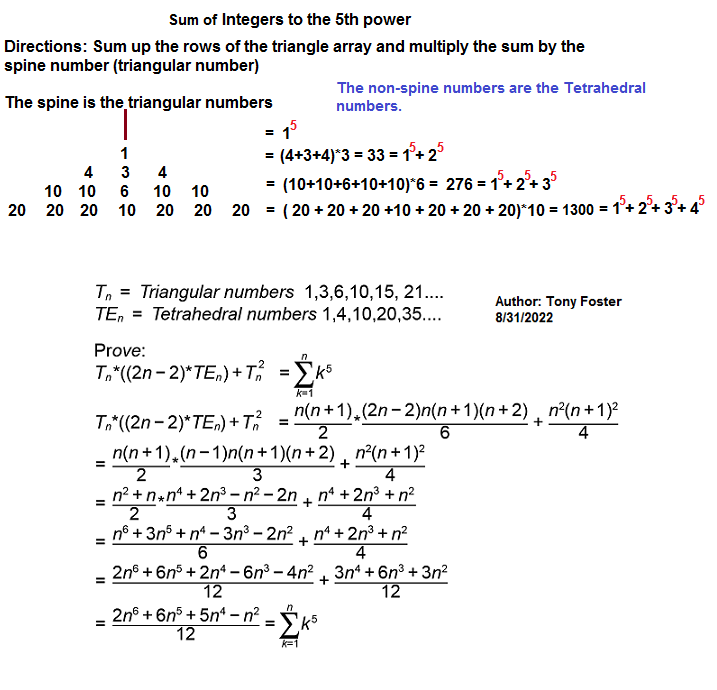(submitted by Tony Foster vide his email dated 31 August 2022).

If you find any new and interesting observation about triangular numbers, please email me.

---------------------------------------------------------------------------

 Ball, W. W. R. and Coxeter, H. S. M. Mathematical Recreations and Essays, 13th ed. New York: Dover, p. 59, 1987.

 Beiler, Albert H. Recreations in the Theory of Numbers. New York: Dover, 1966.

 Conway, J. H. and Guy, R. K. The Book of Numbers. New York: Springer-Verlag, pp. 33-38, 1996.

 Dudeney, H. E. Amusements in Mathematics. New York: Dover, pp. 67 and 167, 1970.

 Gupta, Shyam Sunder "Curious Properties of triangular numbers." Science Reporter , September 1987, India.

 Guy, R. K. Unsolved Problems in Number Theory, 2nd ed. New York: Springer-Verlag, pp. 136-138 and 147-150, 1994.

 Le Lionnais, F. Les nombres remarquables. Paris: Hermann, 1983.

 Pickover, Clifford A. Wonders of Numbers. New York: Oxford University Press, 2001.

 Sloane, N. J. A. and Plouffe, S. The Encyclopedia of Integer Sequences. San Diego, CA: Academic Press, 1995.

 Trotter, T. Jr. "Some Identities for the Triangular Numbers." J. Recr. Math. 6, 128-135, 1973.

 Wells, David. The Penguin Dictionary of Curious and Interesting Numbers. Middlesex, England: Penguin Books, 1997.

 Wells, David. The Penguin Dictionary of Curious and Interesting Geometry. London: Penguin Books, 1991.

 Gupta, Shyam Sunder "Smarandache Sequence of Triangular Numbers", Smarandache Notions Journal, (Vol. 14, 2003).